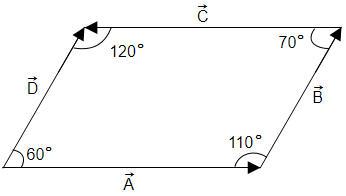# NEET Physics Mathematical Tools Questions Solved

In the given figure(A)  Angle between $\stackrel{\to }{\mathrm{A}}$ and $\stackrel{\to }{\mathrm{B}}$ is $110°$

(B)  Angle between $\stackrel{\to }{\mathrm{C}}$ and $\stackrel{\to }{\mathrm{D}}$ is $60°$

(C)  Angle between $\stackrel{\to }{\mathrm{B}}$ and $\stackrel{\to }{\mathrm{C}}$ is $110°$

(D)  Angle between $\stackrel{\to }{\mathrm{B}}$ and $\stackrel{\to }{\mathrm{C}}$ is $70°$